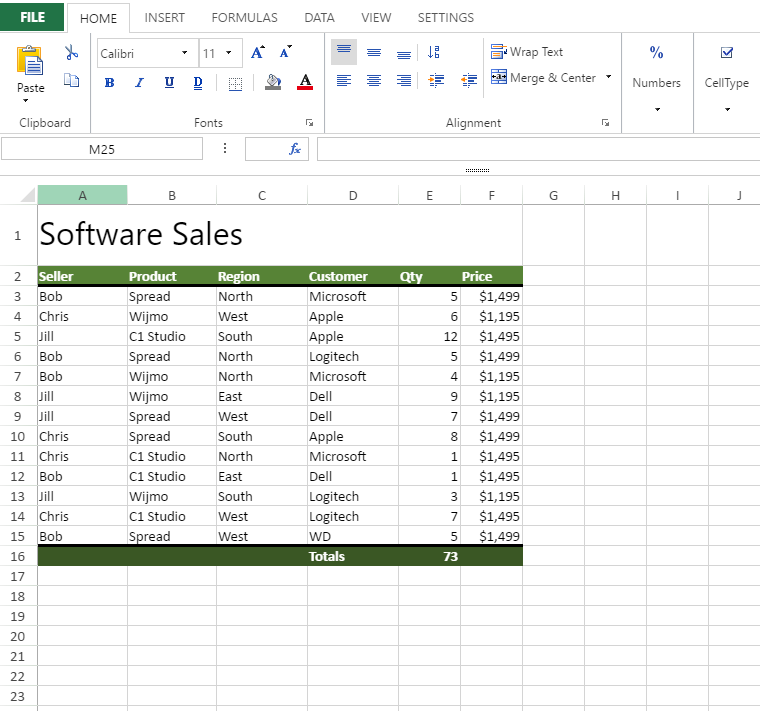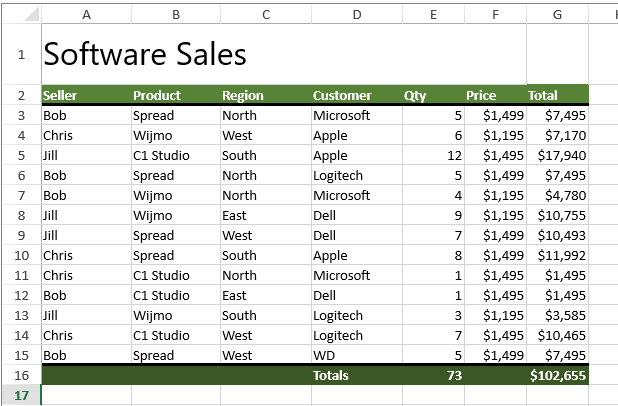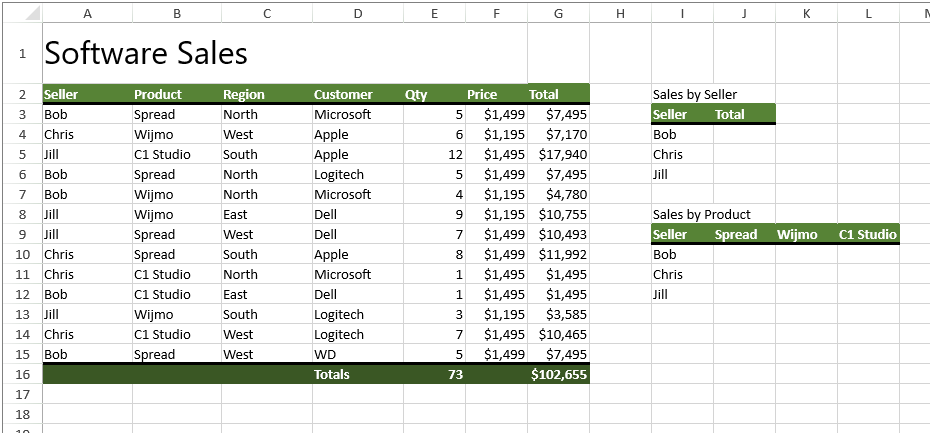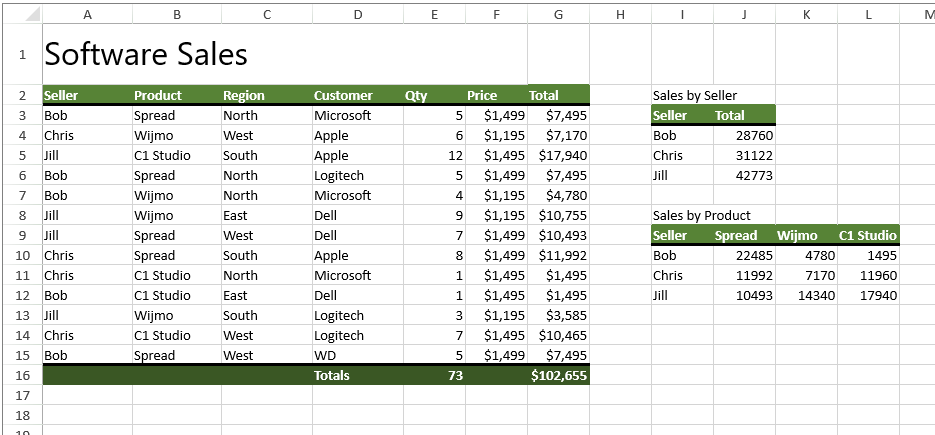﻿ Simplifying Calculations Using Excel Array Formulas in a JavaScript Spreadsheet | Spread# Simplifying Calculations Using Excel Array Formulas in a JavaScript Spreadsheet

Array formulas are one of the most powerful and underused calculation features in a spreadsheet, allowing users to replace thousands of formulas with simpler calculations while providing the same result. They can be used to easily create, for example, another automatically calculated column in a sales table or to create some filtered data. A few benefits of using array formulas include:

• Eliminating the need for intermediate formulas in complex calculations
• Some calculations are impossible without array formulas, as they need to use entire arrays of values rather than a single value
• Can give a single result or multiple results
• Ensures consistency and speed, as the arrays used in the formulas would be stored in memory

Array formulas can do so much more, but this tutorial will focus on these simple examples to get you started with array formulas in SpreadJS.We can start by adding the script/css references and initializing the Spread instance:

``````<!DOCTYPE html>
<html xmlns="http://www.w3.org/1999/xhtml">
<meta charset="utf-8" />
<title>SJS Array Formulas</title>

<script type="text/javascript" src="ArrayFormulaTest.js"></script>

<script>

}
</script>
<body>
<div id="spreadSheet" style="width: 825px; height: 800px; border: 1px solid gray"></div>
</body>
</html>
``````

``````spread.fromJSON(ArrayFormulaTest);

``````

## Add Table Column with Array Formula

The first array formula we will add is going to be to create a total column. Normally, we would create a formula for each cell to multiply the quantity column by the price column. Rather than creating a formula to fill, we can create an array formula that does this work:

=E3:E15*F3:F15

This will return an array of values rather than a single value, which will be applied to the entire column. Setting an array formula in SpreadJS requires defining the row, column, row count, column count, and formula, like so:

``````activeSheet.setArrayFormula(2, 6, 13, 1, "E3:E15*F3:F15");
``````

We can then format that new column:

``````spread.options.allowUserEditFormula = true;
activeSheet.getCell(1, 6).value("Total");
activeSheet.setFormula(15, 6, "SUM(G3:G15)");
activeSheet.getRange(1, 6, 15, 1).formatter("\$#,#");

``````Using an array formula for this drastically reduces the amount of formulas that would normally be needed to create a total column for each row.

## Summarize with Array Formulas

Another use of array formulas is to more easily summarize data. For example, you may want to add together values only if they meet certain conditions, something that can't be done easily with normal formulas. Typically you would use an IF statement, which, when applied to a range of cells, would return an array of positive and false values. An array formula would be the only way to effectively use this in a single cell. We can make two tables for this: Sales by Seller and Sales by Product for each seller:

``````activeSheet.getCell(1, 8).value("Sales by Seller");
activeSheet.getCell(2, 8).value("Seller");
activeSheet.getCell(2, 9).value("Total");
activeSheet.getCell(3, 8).value("Bob");
activeSheet.getCell(4, 8).value("Chris");
activeSheet.getCell(5, 8).value("Jill");

activeSheet.getCell(7, 8).value("Sales by Product");
activeSheet.getCell(8, 8).value("Seller");
activeSheet.getCell(8, 10).value("Wijmo");
activeSheet.getCell(8, 11).value("C1 Studio");
activeSheet.getCell(9, 8).value("Bob");
activeSheet.getCell(10, 8).value("Chris");
activeSheet.getCell(11, 8).value("Jill");
``````We can start with the Sales by Seller table. In this instance, we will add all of the sales for each specific seller using an array formula. To make the formula simpler, we can go ahead and add some custom names to use:

``````activeSheet.addCustomName("Seller", "\$A\$3:\$A\$15", 0, 0);
``````

Then we define the array formula like so:

=SUMIF(Seller, I4:I6,Total)

We can then set that array formula in all three cells at once:

``````activeSheet.setArrayFormula(3, 9, 3, 1, "SUMIF(Seller, I4:I6,Total)");
``````

The array formulas in the Sales by Product table are going to be similar, but in this case we are going to add up each seller's totals by the products that they sold, which can be done with an array formula like so:

=SUMIFS(Total, Seller, \$I\$10:\$I\$12, Product, \$J\$9:\$L\$9)

This will be defined for each cell in the table:

``````activeSheet.addCustomName("Product", "\$B\$3:\$B\$15", 0, 0);
activeSheet.setArrayFormula(9, 9, 3, 3, "SUMIFS(Total, Seller, \$I\$10:\$I\$12, Product, \$J\$9:\$L\$9)");

``````These are just a couple of simple examples of array formulas, but they can be used for so much more. Now that array formulas are supported in SpreadJS, you can easily import your most advanced Excel files. The possibilities for even more advanced Excel functionality in your spreadsheets are endless.Kevin Ashley

Product Manager